TWiki>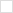WebXEO Web>XeoPrimer>XeoPrimerBOL (2011-04-04, NicolauGrosskopf)
Tags:
create new tag
, view all tags
-- PedroRio - 21 Dec 2010

The Business Object Language is a small expression language to help define conditions for the behavior in XEO Object Models. It allows to reference attribute values, compare them with other values as well as making small calculations. The following sections explain the various operators and statements that can be used in the language.

### Attributes

XEO Model attributes can be referenced in BOL expressions by their name, for example if you want to create an expression that represents the following ideia: "If the value of attribute X is bigger than 5, return true" the bol expression would be "if (x > 5) return true". This syntax works for attributes that are not Collection nor Object.

When dealing with a XEO Model attribute you can refer to the values of its attributes by using the separator '.' ; Imagine a situation where we have a XEO Model to represent a Person, a Car and a Car Brand, where Person has 1:1 relation to Car which has a 1:1 relation to Brand, those relations are depicted in figure BOL.1. The following tables have the definition of the Object Models.

Person ( Person )

Attribute Name Attribute Type Label Description Length Required Object Type
name Text Name Person Name 30 - N/A
avg_km_car_year Number Average Km's Average KM's per year with a car 30 - N/A
ID Text Identifier The identifier of the person 30 - N/A
age Number Age The age of the person 3 - N/A
favorite_car Text Favorite Car The favorite car of the person 30 - N/A
car Object Car The car of the person 30 - Car

Car ( Car )

Attribute Name Attribute Type Label Description Length Required Object Type
model Text Model The model of the car 30 - N/A
age Number Age The age of the car 3 - N/A
kms Number Kilometers The number of kilometers the car has done 4 - N/A
brand Object Brand The car brand 4 - N/A

Brand ( Brand )

Attribute Name Attribute Type Label Description Length Required Object Type
name Text Name Name of the category 30 true N/A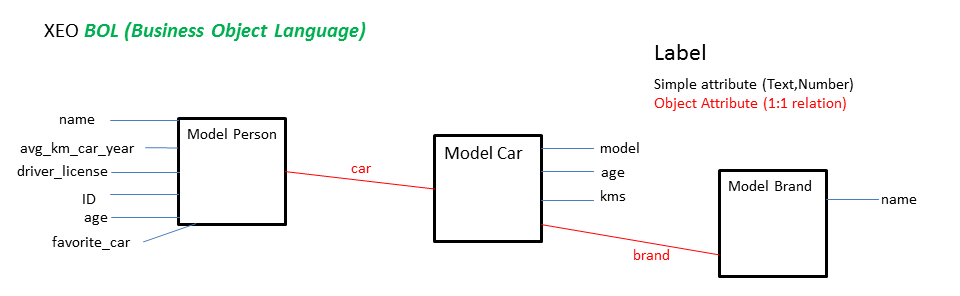Figure BOL.1 - XEO Models for the BOL example.

Tentar verificar se é possível usar "parent.att" em BOL `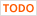`

### Boolean Values

Boolean values can be used in BOL expressions. The folowing expressions represent the "true" value:

• true
• yes
• y
• YES

In the same way, the following values represent the "false" value:

• false
• no
• n
• NO

### Conditional Statements

In BOL you can use conditional expressions in the form of if/else statements. The syntax is like the following:

```if ( CONDITION )
BOL EXPRESSION
else
BOL EXPRESSION```

If you need more conditions you use if/else statements with brackets

```if (CONDITION ){
//BOL EXPRESSIONS
//BOL EXPRESSIONS
} else {
//BOL EXPRESSIONS
//BOL EXPRESSIONS
}
```

_

### Returning a value

Most BOL expressions are used in places where they must return a value (be it a boolean, string or numeric value). In order to do that BOL has the expression "return" which will return the result of evaluating the expression on its right side. Example:

```return true

```
```return YES

---

if (age > 18)
return "You can drive"
else
return "Need to wait a little longer"```

### Operators

BOL expressions can have multiple operands linked by operators (as with most programming languages). In BOL the following operators are allowed:

• > (Bigger)
• >= (Bigger or Equal)
• == (Equal)
• <= (Less or Equal)
• < (Less)
• ! (Negation)
• && ( And)
• || (Or)
• ! = (Different)

You can have expressions such as:

```if (att1 > 2 && att2 != 45 && att3 == 'F') return "42"
```

_

### Functions

BOL includes a set of predefined functions which can be used inside every BOL Expression.

DATE FUNCTIONS

NOW - Returns the current date with time

TODAY - Returns the current date without time information

NOW_HOURS - Returns the current date with time information about the hour (discards minutes, seconds and mili-seconds)

NOW_MINUTES - Returns the current date with time information about the hour and minutes (discards seconds and mili-seconds)

ROUND

ROUND(Number) - Rounds to the nearest integer (0.4 -> 0; 0.5 -> 1)

CONCAT (Concatenates two values)

• CONCAT(String,String)
• CONCAT (Number,Number) - Number can be long/int value
• CONCAT (Object,Object) - Converts each Object to string and concatenates

TO_DATE (Converts to a Date value)

• TO_DATE(Date, Format)
• TO_DATE (Object, Format), Object is converted to a String and converted to date
• TO_DATE (long, Format) - Convertes UNIX time miliseconds to a date

Format must be compatible with the ones accepted by SimpleDateFormatter (if the format is not correct it will simply return a null value)

The first argument can be one of the following functions (NOW, TODAY, NOW_MINUTES, NOW_HOURS, or any other valid date).

SUM

• SUM ( Number , Number )
• SUM (Object , Object) - Converts the Object to Numbers and sums the numbers

Example:

```Sum(3 , 1 ) will return 4

Sum (att1, att2 )  - assuming both are attributes which contain a value that can converted  to a number will return the sum of the two
```

SUBTRACT

• SUBTRACT (Number, Number)
• SUBTRACT (Object, Object) - Converts the Object to String

Example:

```Substract (3 , 1 ) will return 2

Subtract (att1, att2 ) - assuming both are attributes which contain a value that can converted to a number will return the difference between the two
```

DIFF_IN_DAYS (Difference in days between two dates)

Returns the number of days between two dates

• DIFF_IN_DAYS (Date, Date)
• DIFF_IN_DAY (Date, Long)
• DIFF_IN_DAY (Long, Date)
• DIFF_IN_DAY (Long, Long)

Example:

`DIFF_IN_DAYS (NOW, TO_DATE('22/12/2012','dd/MM/yyyy')) which will return the number of days between today and December 22nd, 2012`
```DIFF_IN_DAYS (TO_DATE('20/12/2012','dd/MM/yyyy'), TO_DATE('22/12/2012','dd/MM/yyyy')) which will return 2 (two)
```

DIFF_IN_UTIL_DAYS (Difference in work days between two dates)

• DIFF_IN_UTIL_DAYS (Date, Date)
• DIFF_IN_UTIL_DAYS (Date, Long) - The long value is a Unix timestamp
• DIFF_IN_UTIL_DAYS (Long, Date) - The long value is a Unix timestamp
• DIFF_IN_UTIL_DAYS (Long, Long) - The long value is a Unix timestamp

IS_NULL (Checks if a given value is null)

Works for all kinds of attributes except for Collection attributes. Returns true is the value of the attribute is null (i.e. newly created object will have all attributes null (except if they have a default value)).

Example:

`if (IS_NULL (att1)) return true`

_

### Examples

This section shows some examples of BOL expressions using the previous Model (Person, Car and Brand)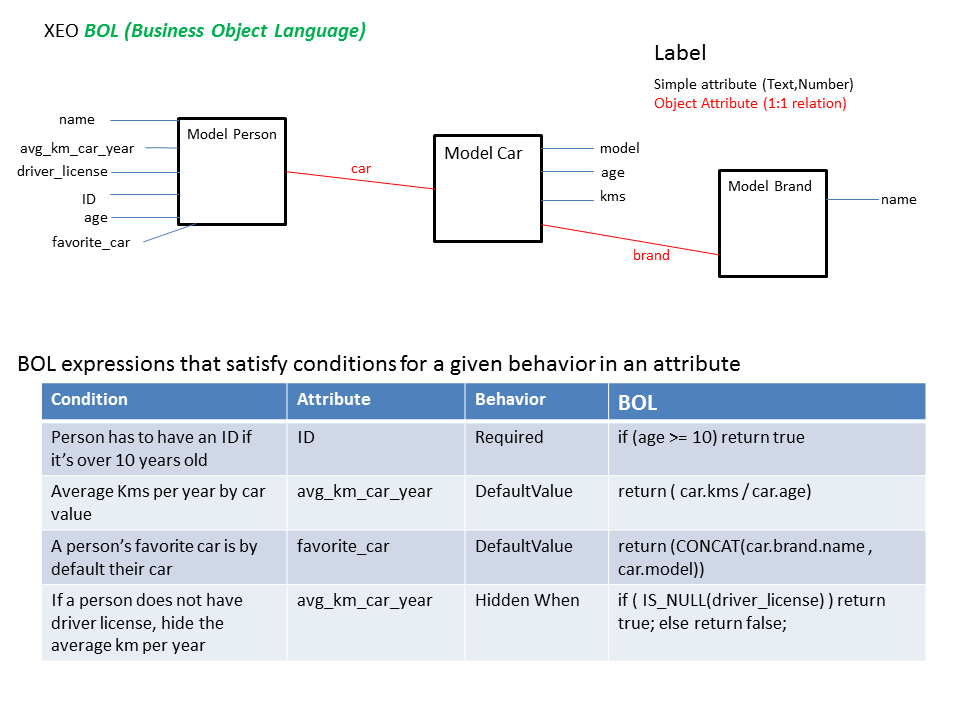Figure BOL.2 - Examples of BOL Expressions

_

Topic attachments
I Attachment Action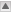Size Date Who Comment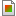png BOL.png manage 8.1 K 2010-12-22 - 15:59 PedroRiopng BOL_Complete.png manage 33.0 K 2010-12-22 - 16:43 PedroRio BOL Complete
Topic revision: r12 - 2011-04-04 - NicolauGrosskopf

No permission to view TWiki.WebTopBar